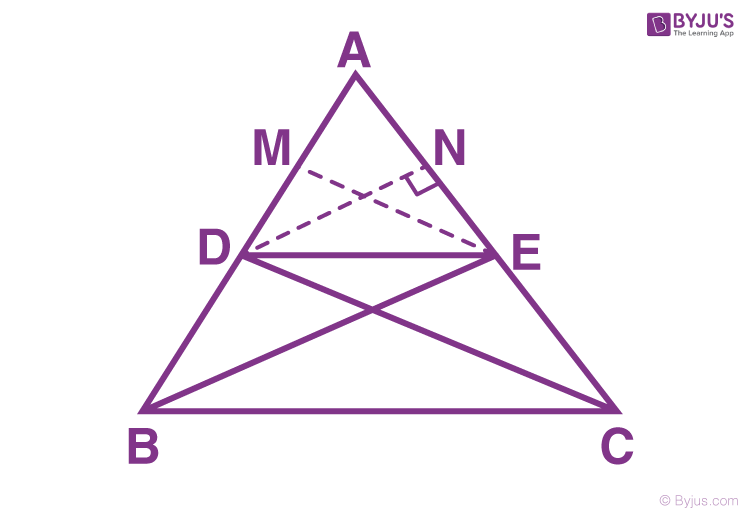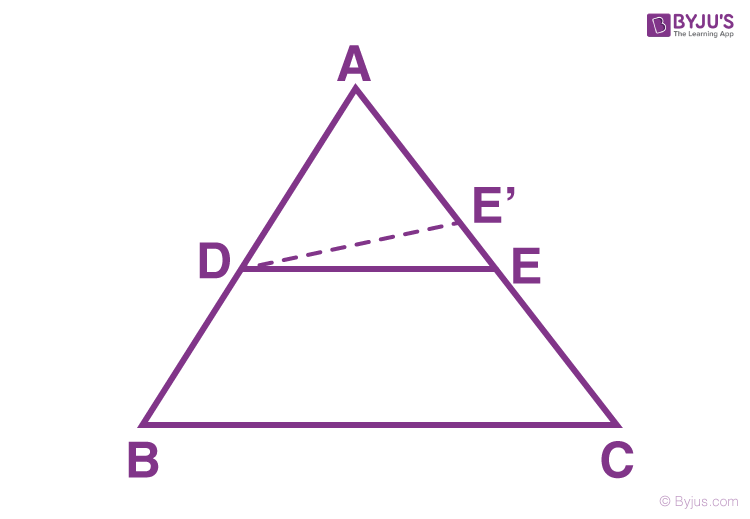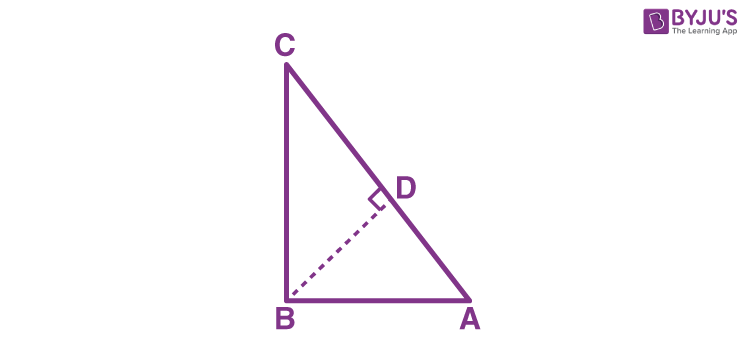# Maths Theorems

There are several maths theorems which govern the rules of modern mathematics. Almost in every branch of mathematics, there are numerous theorems established by renowned mathematicians from around the world. Here, the list of most important theorems in maths for all the classes (from 6 to 12) are provided, which are essential to build a stronger foundation in basic mathematics.

To consider a mathematical statement as a theorem, it requires proof. The proof confirms that the given mathematical statement is true.  Proving the theorems develops logical thinking and reasoning skills along with avoiding Maths errors.

## What are Theorems in Maths?

Mathematical theorems can be defined as statements which are accepted as true through previously accepted statements, mathematical operations or arguments. For any maths theorem, there is an established proof which justifies the truthfulness of the theorem statement.

### Why are Theorems Important in Maths?

Theorems are of significance and are considered as absolute truths. Theorems not only help to solve mathematical problems easily but their proofs also help to develop a deeper understanding of the underlying concepts. For students, theorems not only form the foundation of basic mathematics but also helps them to develop deductive reasoning when they completely understand the statements and their proofs.

## Maths Theorems for Class 10

In Class 10 Maths, several important theorems are introduced which forms the base of mathematical concepts. Class 10 students are required to learn thoroughly all the theorems with statements and proofs, not only to score well in the board exam but also to create a stronger foundation in the subject. Some important maths theorems for Class 10 are listed below.

### List of Important Class 10 Maths Theorems

For Class 10, some of the most important theorems are:

• Pythagoras Theorem
• Midpoint Theorem
• Remainder Theorem
• Fundamental Theorem of Arithmetic
• Angle Bisector Theorem
• Inscribed Angle Theorem
• Ceva’s Theorem
• Bayes’ Theorem

Apart from these theorems, the lessons that have the most important theorems are circles and triangles. Some important triangles and circles theorems for 10th standard are given below.

## Circle Theorems for Class 10

There are various theorems related to the circle. The circle theorems are important for both Class 9 and 10 students. A few important theorems are:

• Theorem 1: Equal chords of a circle subtend equal angles, at the centre of the circle.
• Converse of Theorem 1: If two angles subtended at the centre, by two chords are equal, then the chords are of equal length.
• Theorem 2: The perpendicular to a chord, bisects the chord if drawn from the centre of the circle.
• Converse of Theorem 2: A straight line passing through the centre of a circle to bisect a chord, is perpendicular to the chord.
• Theorem 3: Equal chords of a circle are equidistant (equal distance) from the centre of the circle.
• Converse of Theorem 3: Chords of a circle, which are at equal distances from the centre, are equal in length.
• Theorem 4: Measure of angles subtended to any point on the circumference of the circle from the same arc is equal to half of the angle subtended at the centre by the same arc.
• Theorem 5: The opposite angles in a cyclic quadrilateral are supplementary.

## Triangles Theorems for Class 10

• All congruent figures are similar, but it doesn’t mean that all similar figures are congruent.
• Two polygons with the same number of sides are similar if their corresponding angles are equal, and their corresponding sides are in the same ratio.
• Two triangles are similar if their corresponding angles are equal and corresponding sides are in the same ratio.
• In two triangles, if the corresponding angles are equal and corresponding sides are in the same ratio then the two triangles are similar.
• In two triangles, if the sides of any one of the triangles are proportional to the sides of the other triangle, then their corresponding angles are equal, and the two triangles are similar.

## Proofs

Theorem 1:

If a line is drawn parallel to one side of a triangle and intersects the other two sides, then the other two sides are divided in the same ratio.

Construction: ABC is a triangle, DE is a line parallel to BC and intersecting AB at D and AC at E, i.e. DE || BC.

Join C to D and B to E. Draw EM ⊥ AB and DN ⊥ AC.We need to prove that AD/DB = AE/EC

Proof:

Similarly,

Ar(BDE) = ½ × DB × EM

Ar(ADE) = ½ × AE × DN

Ar(DEC) = ½ × EC × DN

Hence,

Similarly,

Triangles DEC and BDE are on the same base, i.e. DE and between same parallels DE and BC.

Hence,

Ar(BDE) = Ar(DEC)

From the above equations, we can say that

Hence, proved.

Theorem 2:

If a line divides any of the two sides of a triangle in the same ratio, then that line is parallel to the third side.

ABC is a triangle in which DE divides AC and AB in the same ratio. This states that:

AB/DB = AE/ECConstruction: Draw a line DE’ from point D to E’ at AC. Let us consider DE’//BC.

Proof:

To prove: If DE || BC, then

AB/DB = AE/EC

According to the theorem, AB/DB = AE/EC

Then, accordingly, E and E must be coincident.

This proves that DE || BC

Proved.

Theorem 3:

In two triangles, if the sides of one triangle are proportional to the sides of the other triangle, then their corresponding angles are equal and hence the two triangles are similar. It also called SSS (side-side-side) criterion.

Two triangles ABC and DEF are drawn in such a way that their corresponding sides are proportional. It means:

AB/DE = AC/DF = BC/EF

Proof:

To prove: ∠A = ∠C, ∠B = E and ∠C = ∠F

Hence, triangle ABC ~ DEF

In Triangle DEF, draw a line PQ so that DP = AB and DQ = AC

Since the corresponding sides of the two triangles are equal.

This implies;

DP/PE = DQ/QF = PQ/EF

This also means that ∠P = ∠E and ∠Q = ∠F

We had taken, ∠A=∠D, ∠B=∠P and ∠C=∠Q

Hence,

∠A = ∠D, ∠B = ∠E and ∠C = ∠F

Therefore, from AAA criterion;

Triangle ABC ~ DEF.

Proved.

Theorem 4:

Pythagoras Theorem: In a right-angled triangle, the square of the hypotenuse is equal to the sum of the squares of the other two sides.

Hypotenuse2 = Base2 + Perpendicular2

Proof:

ABC is a triangle which is right-angled at B. BD is perpendicular to hypotenuse AC drawn from vertex B.To prove: AC2 = AB2 + BC2

AB2 = AC × AD ……………(1) [Since these are similar triangles.]

In triangles ABC and BDC;

BC/AC = CD/BC

BC2 = AC × CD ………….(2)

When we add 1 and 2, we get;

AB2 + BC2 = AD × AC + CD × AC

AB2 + BC2 = AC (AD + CD)

AB2 + BC2 = AC2

Hence, proved.